# Olympiad Test: Figure Matrix - 1

## 10 Questions MCQ Test Mathematical Olympiad Class 8 | Olympiad Test: Figure Matrix - 1

Description
Attempt Olympiad Test: Figure Matrix - 1 | 10 questions in 20 minutes | Mock test for Class 8 preparation | Free important questions MCQ to study Mathematical Olympiad Class 8 for Class 8 Exam | Download free PDF with solutions
QUESTION: 1

### Direction: In the following questions, study the given matrix, and find the correct option for the question mark form the given four options.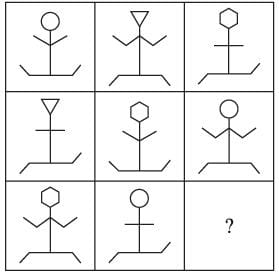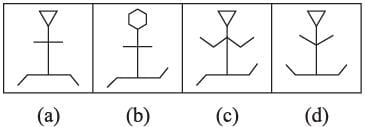Solution:

There are there types of faces, hands and legs. Each type is used once in each row.

QUESTION: 2

### Direction: In the following questions, study the given matrix, and find the correct option for the question mark form the given four options.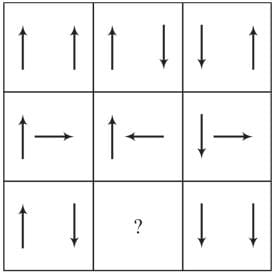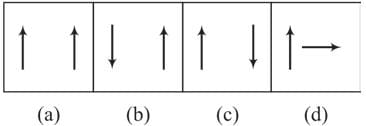Solution:

The 2nd figure is obtained from the first figure by reversing the direction of RHS arrow. and 3rd figure is obtained from second figure by reversing the direction of each arrow.

QUESTION: 3

### Direction: In the following questions, study the given matrix, and find the correct option for the question mark form the given four options.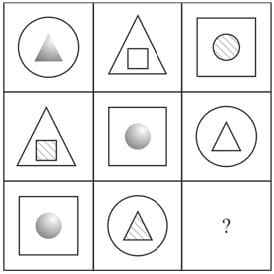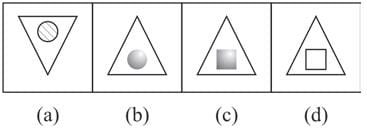Solution:
QUESTION: 4

Direction: In the following questions, study the given matrix, and find the correct option for the question mark form the given four options.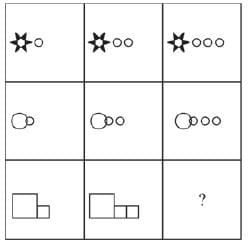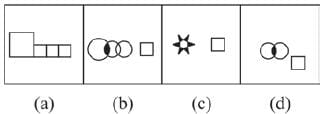Solution:
QUESTION: 5

Direction: In the following questions, study the given matrix, and find the correct option for the question mark form the given four options.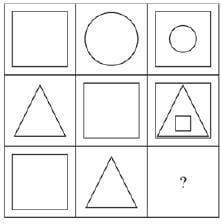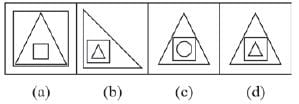Solution:
QUESTION: 6

Direction: In the following questions, study the given matrix, and find the correct option for the question mark form the given four options.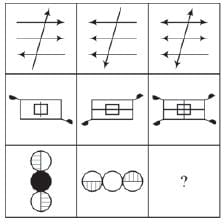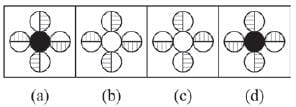Solution:
QUESTION: 7

Direction: In the following questions, study the given matrix, and find the correct option for the question mark form the given four options.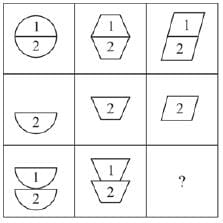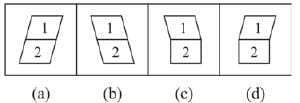Solution:
QUESTION: 8

Direction: In the following questions, study the given matrix, and find the correct option for the question mark form the given four options.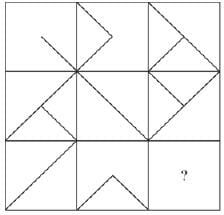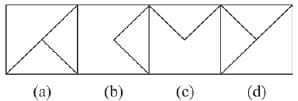Solution:

The 3rd figure in each row consists of parts which are not common in first two figure.

QUESTION: 9

Direction: In the following questions, study the given matrix, and find the correct option for the question mark form the given four options.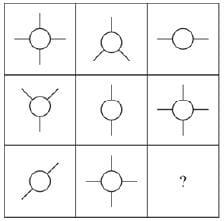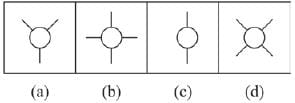Solution:
QUESTION: 10

Direction: In the following questions, study the given matrix, and find the correct option for the question mark form the given four options.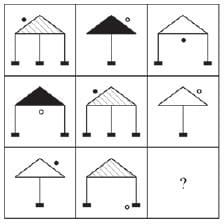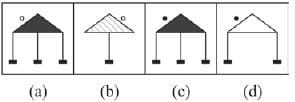Solution:

There are three types of shading in the triangle, three types of legs, three position of circles, each of which is used only once.Use Code STAYHOME200 and get INR 200 additional OFF Use Coupon Code